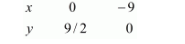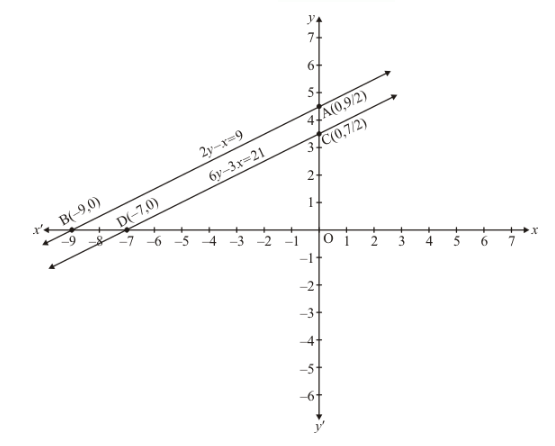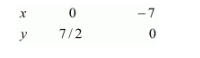# Show graphically that each one of the following systems of equations is in-consistent (i.e. has no solution) :

Question:

Show graphically that each one of the following systems of equations is in-consistent (i.e. has no solution) :

$2 y-x=9$

$6 y-3 x=21$

Solution:

The given equations are

$2 y-x=9$$.(i)$

$6 y-3 x=21$(ii)

Putting $x=0$ in equation $(i)$, we get:

$\Rightarrow 2 y-0=9$

$\Rightarrow y=9 / 2$

$\Rightarrow x=0, \quad y=9 / 2$

Putting $y=0$ in equation $(i)$ we get:

$\Rightarrow 2 y-0=9$

$\Rightarrow y=9 / 2$

$\Rightarrow x=0, \quad y=9 / 2$

Putting $y=0$ in equation $(i)$ we get:

$\Rightarrow 2 \times-x=9$

$\Rightarrow x=-9$

$\Rightarrow x=-9, \quad y=0$

Use the following table to draw the graph.Draw the graph by plotting the two points $A(0,9 / 2), B(-9,0)$ from table.$6 y-3 x=21$ $\ldots . .(i i)$

Putting $x=0$ in equation $(i i)$ we get:

$\Rightarrow 6 y-3 \times 0=21$

$\Rightarrow y=7 / 2$

$\Rightarrow x=0, \quad y=7 / 2$

Putting $y=0$ in equation $(i i)$, we get:

$\Rightarrow 6 \times 0-3 x=21$

$\Rightarrow x=-7$

$\therefore x=-7, \quad y=0$

Use the following table to draw the graph.Draw the graph by plotting the two points $C(0,7 / 2), D(-7,0)$ from table.

Here two lines are parallel and so don’t have common points

Hence the given system of equations has no solution.